# The Heat Transfer Study in the Diesel Engine Combustion Chamber Using a Two-Zone Combustion Model

The Heat Transfer Study in the Diesel Engine Combustion Chamber Using a Two-Zone Combustion Model

Brahim MenacerNaima Khatir Mostefa Bouchetara Ahmed Amine Larbi Cherif Belhout

Higher School of Electrical Engineering and Energetics ESGEE Oran, BP 64 CH2, Achaba Hanifi -USTO, Oran 31000, Algeria

Science Faculty, BP: 66, University Centre of Naama, Naama 45000, Algeria

Laboratory of Gas Combustion and Environment, Department of Mechanical Engineering, University of Sciences and the Technology of Oran, L.P 1505 El -Menaouer, USTO, Oran 31000, Algeria

Renewable Energies Research Unit in the Saharan Environment, URERMS, Renewable Energies Development Center, CDER, Adrar 01000, Algeria

Corresponding Author Email:
menacer_brahim@esgee.dz
Page:
614-620
|
DOI:
https://doi.org/10.18280/mmep.070414
Received:
27 July 2020
|
Accepted:
12 October 2020
|
Published:
18 December 2020
| Citation

OPEN ACCESS

Abstract:

The study of heat transfer phenomena in diesel engines is a very complex task considering the number of engine components such as intake and exhaust manifolds, lubricant oil and coolant subsystems, the different heat transfer mechanisms (conduction, convection, and radiation). This paper presents simulation results using a dual-zone model associated to GT-Suite simulation software for the calculation of convective heat transfer from gas to the cylinder wall, radiation heat transfer, gas pressure and temperature for low, partial and full load engine as a function of crank angle for a single-cylinder diesel engine. In this present article, a numerical simulation model was created to foresee the main combustion characteristics, and the simulated results were approved through the reference experiment data. Simulation results showed that any increase in the mass of fuel injected into the combustion chamber would generate a significant increase in the level of pressure and temperature of the combustion gases in the cylinder. This means that despite the improved power performance, excessive fuel consumption would have a negative effect on the thermal behavior and consequently on the life of the engine. The essential objective of any combustion engine development is to reduce fuel consumption while maintaining or improving the engine's power output.

Keywords:

convective heat transfer, radiation heat transfer, Wiebe function, modeling, GT-suite, diesel engine

1. Introduction

For several years, the heat transfer process in diesel engines has become a subject of great importance due to the need to improve engine performances as torque, power, and efficiency, to reduce fuel consumption and pollutant emissions. On the other hand, the loss due to the increase in heat transfer from the burnt gases to the combustion chamber walls lowers the temperature and pressure of the gas inside the cylinder, which reduces the work per cycle transferred to the piston. Fuel-injected into the combustion chamber of a diesel engine loses up to 30% of its energy due to the heat transfer transferred from the working gas to the cooling system. About 50% of this energy is lost by the piston and 30% by the head . Menacer and Bouchetara  studied the main performances of a turbocharged diesel engine using the simulation model and using GT-suite software to validate the results. A good agreement was found between the numerical results in FORTRAN program and GT-suite. Tianyu et al.  perform an experimental test in a constant volume vessel to study the impact of fuel spray on the near-wall combustion. According to these results, the lower ambient temperature makes the circumferential flame non-uniform, and its downstream evolution is sensitive to the temperature of the wall. The combustion and emissions of a direct injection diesel engine were treated in the work of Dimitrios C. Rakopoulos et al. . By examining the effect of oxygenating diesel fuel at different injection angles and loads. It showed an increase in in-cylinder pressure, temperature and NO emissions, and a decrease at any load in soot emissions with the degree of the fuel oxygenation. In the results of Yong Liu et al. , a two-dimensional transient thermal heat conduction model was improved by using a computer simulation program to predict the engine cylinder wall temperatures. This method gave good agreement with the experimental results carried out in two diesel engines, Isuzu and Caterpillar. Yong et al.  developed results of a simulation computer program in a direct injection diesel engine and compare it with tests carried out on a test bench by testing of two modelling combustion approaches (multi-zone model and in-cylinder flow model). A thermodynamic simulation model studied in the paper of Salah et al. , shows its ability to predict the impact of operating conditions on the combustion characteristics of a constant volume engine. CD. Rakopoulos et al.  evaluated the soot formation and oxidation rates in the direct injection diesel engine closed cycle by choosing a dual-zone combustion model. There was a very good agreement between the results from the simulation program and the experimental results carried out on a “Hydra” test bench. Paramust Juntarakod et al.  have carried out numerical and experimental studies on the diesel/ethanol mixture injected into a combustion chamber of diesel engines by developing a multi-zone combustion model. The results show a remarkable increase in maximum performance parameters with increased engine speed and equivalence ratio. Salman Abdu et al.  have shown using GT-Power software that the use of the dual-fuel mode is important for reducing emissions and improving the performance of the turbocharged direct injection diesel engine.

A dual-zone combustion model used in the article of Ivan Arsie et al. , which can predict the effect of the injection parameters (whatever the type of single or multiple injections), in a short calculation time to optimize the control parameters of the HSDI engines.

The main objective of this research paper is to develop a dual-zone combustion simulation model in a direct injection single-cylinder diesel engine and using the GT-Suite software to predict the total, convective and radiation heat transfer, pressure and temperature of the burnt and unburnt gases as a function of the crank angle for different engine loads. In this article, the simulation results have been validated using experimental data from the literature. The two results show a good agreement between them.

2. Model Equations

Certain simplifications to build the dual-zone combustion model have been made through this paper:

• The engine cylinder is divided into dual zones (burnt zone and unburnt zone) and separated by a thin flame, as shown in Figure 1. Each of these zones is assumed to be spatially homogeneous .
• In the compression period, the model is supposed in a single-zone model and undergoes no pre-flame reactions.
• During all the calculations of the engine thermodynamic cycle, the gases are assumed ideal.
• It is assumed that the combustion chamber pressure is the same for both zones (burnt zone and unburnt zone).
• No heat transmission between both zones (burnt zone and unburnt zone) .
• The temperature and pressure of the gases in the intake and exhaust manifolds are assumed to be constant.
• In this study, we do not take into account the crevice losses.
• The instant thermodynamic properties used in this study are taken from JANAF tables .

## 1.jpgFigure 1. Dual-zone combustion model

The dual-zone combustion model uses the following equations (energy conservation, ideal gases, and mass conservation) :

Valve leakage and blow-by are overlooked, so the cylinder total mass is:

$m=m_{b}+m_{u}$     (1)

The total combustion chamber volume is the sum of both zones’ volumes (burnt zone and unburnt zone):

$V=V_{b}+V_{u}$     (2)

The equation of state of the ideal gas in each zone is given by:

$\left\{\begin{array}{l} P \cdot V_{u}=m_{u} \cdot R_{u} \cdot T_{u} \\ P \cdot V_{b}=m_{b} \cdot R_{b} \cdot T_{b} \end{array}\right.$     (3)

The in-cylinder pressure and temperature equations of burned and unburned zones are given by :

$\frac{d P}{d \theta}=\frac{A+B+C}{D+E}$     (4)

\begin{aligned} \frac{d T_{b}}{d \theta}=\frac{-h\left(\pi b^{2} / 2+4 V / b\right) x^{0.5} T_{b}-T_{w}}{v m c_{p, b} x}+\frac{v_{b}}{c_{p, b}}\left(\frac{\partial \ln v_{b}}{\partial \ln T_{b}}\right) \times \\ \left(\frac{d P}{d \theta}\right)+\frac{h_{u}-h_{b}}{x c_{p, b}}\left[\frac{d x}{d \theta}-x-x^{2} \frac{C}{\omega}\right] \end{aligned}     (5)

\begin{aligned} \frac{d T_{u}}{d \theta}=\frac{-h\left(\pi b^{2} / 2+4 V / b\right) x^{0.5} T_{u}-T_{w}}{v m c_{p, u} x}+\frac{v_{u}}{c_{p, u}}\left(\frac{\partial \ln v_{u}}{\partial \ln T_{u}}\right) \times \\ \left(\frac{d P}{d \theta}\right)+\frac{h_{u}-h_{b}}{x c_{p, u}}\left[\frac{d x}{d \theta}-x-x^{2} \frac{C}{\omega}\right] \end{aligned}     (6)

The Olikara and Borman constants are :

$A=\frac{1}{m}\left(\frac{d V}{d \theta}+\frac{V C}{\omega}\right)$     (7)

$B=h \frac{A}{\omega} \times\left[\begin{array}{l} \frac{v_{b}}{c_{p, b}} \frac{\partial \ln v_{b}}{\partial \ln T_{b}} x^{0.5} \frac{T_{b}-T_{w}}{T_{b}}+ \\ \frac{v_{u}}{c_{p, u}} \frac{\partial \ln v_{u}}{\partial \ln T_{u}}\left(1-x^{0.5}\right) \frac{T_{u}-T_{w}}{T_{u}} \end{array}\right]$     (8)

$C=-v_{b}-v_{u} \frac{d x}{d \theta}-\frac{v_{b}}{c_{p, b}} \frac{\partial \ln v_{b}}{\partial \ln T_{b}} \frac{x^{0.5} h_{u}-h_{b}}{T_{b}}\left[\frac{d x}{d \theta}-\frac{\left(x-x^{2}\right) C}{\omega}\right]$     (9)

$D=x\left[\frac{v_{b}^{2}}{T_{b} c_{p, b}}\left(\frac{\partial \ln v_{u}}{\partial \ln T_{u}}\right)^{2}+\frac{v_{b}}{P} \frac{\partial \ln v_{b}}{\partial \ln P}\right]$     (10)

$E=1-x\left[\frac{v_{u}^{2}}{T_{u} c_{p, u}}\left(\frac{\partial \ln v_{u}}{\partial \ln T_{u}}\right)^{2}+\frac{v_{u}}{P} \frac{\partial \ln v_{u}}{\partial \ln P}\right]$     (11)

The mixture state is given by the following equations :

$\frac{d u}{d \theta}=\left(c_{p}-\frac{P v}{T} \frac{\partial \ln v}{\partial \ln T}\right) \frac{d T}{d \theta}-\left(v\left(\frac{\partial \ln v}{\partial \ln T}+\frac{\partial \ln v}{\partial \ln P}\right)\right) \frac{d T}{d \theta}$     (12)

$\frac{d v}{d \theta}=\frac{v}{T} \frac{\partial \ln v}{\partial \ln T} \frac{d T}{d \theta}-\frac{v}{P} \frac{\partial \ln v}{\partial \ln P} \frac{d P}{d \theta}$     (13)

$\frac{d s}{d \theta}=\frac{c_{p}}{T} \frac{d T}{d \theta}-\frac{v}{T} \frac{\partial \ln v}{\partial \ln T} \frac{d P}{d \theta}$     (14)

$\frac{d W}{d \theta}=P \frac{d V}{d \theta}$     (15)

With: cp=(∂h/∂T)p.

3. Heat Transfer Modelling

From Figure 2, the total heat loss term dQ/ is modelled as :

$\begin{array}{l} \frac{d Q}{d \theta}=\frac{-1}{\omega}\left(\frac{d Q_{c, b}}{d \theta}+\frac{d Q_{c, u}}{d \theta}+\frac{d Q_{r}}{d \theta}\right)= \\ \frac{-1}{\omega}\left(\frac{h A_{c}}{\omega}\left[x^{0.5}\left(T_{b}-T_{u}\right)+\left(1-x^{0.5}\right)\left(T_{u}-T_{w}\right)\right]+\frac{d Q_{r}}{d \theta}\right) \end{array}$     (16)

## 2.jpg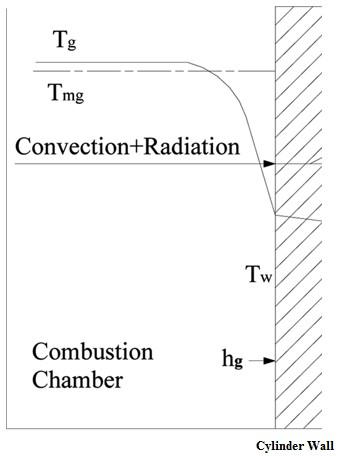Figure 2. In-cylinder heat transfer process in a diesel engine

3.1 Convective heat transfer model

The temperature gradient in the boundary layer influences the convective heat transfer between the gases and the cylinder wall. The convective heat transfer rate is :

$\left\{\begin{array}{l} \frac{d Q_{c, b}}{d \theta}=h \times A_{b} \times\left(T_{b}-T_{\text {wall}}\right) \\ \frac{d Q_{c, u}}{d \theta}=h \times A_{u} \times\left(T_{u}-T_{\text {wall}}\right) \end{array}\right.$     (17)

For the areas Ab and Au, the cylinder area Ac can be divided as follows:

$\left\{\begin{array}{c} A_{c}=\pi b^{2} / 2+4 V / b \\ A_{b}=A_{c} x^{0.5} \\ A_{u}=A_{c}\left(1-x^{0.5}\right) \end{array}\right.$     (18)

The convective heat transfer coefficient used in this paper, calculated by :

$h=3.26 \times D^{-0.2} \times p^{0.8} \times T_{g}^{-0.55} \times w^{0.8}$     (19)

The burned gas velocity can be determined by:

$w(\theta)=2.28 \times \overline{U_{p}}+C_{1} \frac{V_{d} T_{g r}}{p_{r} V_{r}}\left(p(\theta)-p_{m}\right)$     (20)

The motoring pressure (pm) can be calculated from the Watson-Janota model . The values of C1 and C2 depending on the operation period of the engine cycle: compression period (C1=2.28, C2=0), combustion and expansion periods (C1=2.28, C2=3.24×10-3), and exchange period (C1=6.18, C2=0).

The average piston speed $\overline{U_{p}}$ is calculated from :

$\overline{U_{p}}=\frac{2 \times N \times S}{60}$     (21)

3.2 Radiation heat transfer model

In diesel engines, the high-burned gas temperature and soot can be considered as the main sources of thermal radiation. Using the relationship of Anands , the heat transfer by radiation is calculated as a function of the average temperature of the bulk gases :

$\frac{d Q_{r}}{d \theta}=k_{r} \times A \times\left(T_{g}^{4}-T_{w}^{4}\right)$     (22)

The radiation heat flux is neglected during the intake, compression and exhaust periods. For the combustion period, the formula of Anands has been used :

$k_{r}=C_{r} \times \sigma$     (23)

The instantaneous radiant heat flux is evaluated by the following equation:

$Q_{r}=\varepsilon_{a} \times \sigma \times A \times\left(T_{r}^{4}-T_{w}^{4}\right)$     (24)

Using both values (adiabatic flame temperature and average bulk gas temperature) to calculate the value of the apparent radiant temperature :

$T_{r}=\frac{T_{g}+T(\phi=1.1)}{2}$     (25)

For an equivalence ratio of 1.1, the combustion gas temperature is a function of instantaneous air temperature and pressure. This value can be evaluated using the Nasa equilibrium program. Satisfactory accuracy was obtained by considering two temperature ranges :

• For $800 K \prec T_{\text {air}} \prec 1200 K$

$\begin{array}{r} T(\phi=1.1)=\left[1+0.0002317\left(T_{a i r}-950\right)\right] \times \\ \left(2726.3+0.9306 . p-0.003233 . p^{2}\right) \end{array}$     (26)

• For $450 K \prec T_{\text {air}} \prec 800 K$

$\begin{array}{l} T(\phi=1.1)=\left[1+0.000249\left(T_{\text {air}}-650\right)\right] \times \\ \left(2497.3+4.7521 . p-0.11065 . p^{2}+0.000898 \cdot p^{3}\right) \end{array}$     (27)

The instantaneous air temperature (Tair) is calculated from the condition at the start of combustion (ing) :

$T_{a \dot{r}}=T_{a i r, i n g} \times\left(\frac{p}{p_{i n g}}\right)^{\frac{\gamma-1}{\gamma}}$     (28)

The coefficient of apparent grey-body emissivity is varying linearly between its maximal values (0.9) and zero after finished the combustion process [13, 14]:

$\varepsilon_{a}(t)=0.9 \times\left(1-\frac{\theta-\theta_{i n g}}{\theta-\theta_{e v o}}\right)$     (29)

4. Simulation Results

Table 1 present the use of engine specifications and operating conditions in this article to the results' simulation for a single-cylinder direct injection diesel engine.

Table 1. Engine specifications

 Parameters Values Units Bore 119 mm Stroke 87.5 mm Connecting road 300 mm Compression ratio 17:1 - Cylinders number 01 - Injection timing 10° BTDC Intake valve opens 11° BTDC Intake valve closes 32° ABDC Exhaust valve opens 35° BBDC Exhaust valve closes 16° ATDC

Figure 3 presents the validation curves, between the in-cylinder simulated pressure as a function of the crankshaft angle using the dual-zone model and the pressure curve measured from the test bench of a diesel engine used by Maniniyan and Sivaprakasam . A good coincidence is observed between the numerical and experimental pressure, as shown in Figure 3. The error is estimated to be in 3% between them.

## 3.png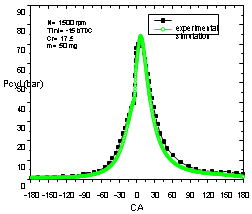Figure 3. Comparison between simulated and experimental in-cylinder pressure versus crank angle

From Figure 4, for fixed engine speed (value of 2,000 rpm), the engine load has a large effect on the in-cylinder pressure, we see a rapid increase in the slope at the point of combustion start (10° before TDC), the pressure peak is at a crankshaft angle of about 12° after TDC. This is may due to the advanced combustion onset of the injected mass fuel. Figure 4 also shows that when the amount of fuel injected into the combustion chamber increases by 50%, the cylinder pressure peak increases by 20%.

## 4.png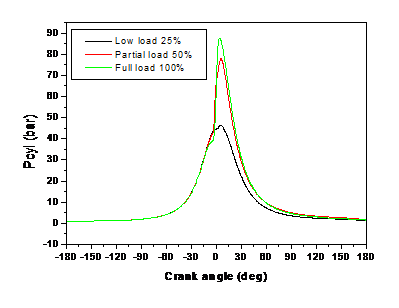Figure 4. Effect of engine load on cylinder pressure versus crank angle

Figure 5 shows the evolution of in-cylinder gas temperature versus crank angle for a low, partial and full load at a fixed engine speed of 2000 rpm, a rapid increase in the slope of temperature at the point where combustion starts (5° before TDC), the peak of temperatures is approximately 35°, 10° and 13° CA after TDC for a low, partial and full load. If the mass fuel-injected increased by 50% (from partial to full load), the maximum cylinder temperature increased by 28 %. The reason behind this is that at full load there is more fuel in the combustion chamber, so the combustion time increases and after that the temperature increases too.

## 5.png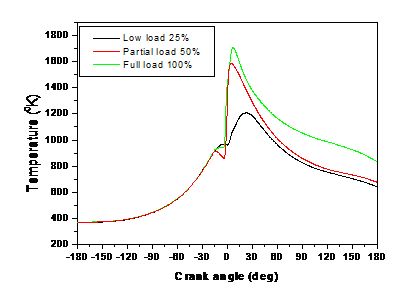Figure 5. Effect of engine load on cylinder temperature versus crank angle

Figure 6 represents the variation of the in-cylinder gas temperature as a function of the crankshaft angle in the two zones (burnt and unburnt), engine speed of 2,000 rpm, injection timing angle of 15 BTDC and for two engine loads (full load and partial load). A very rapid increase in temperature of the burning zone with the start of combustion is depicted. The high burning zone temperature is responsible for the formation of NO and soot. And on the other hand, a slight change in temperature in the unburned zone. The peak temperature is 1,700K, 1,600K and 1,300K at respectively full, partial and low load.

## 6.png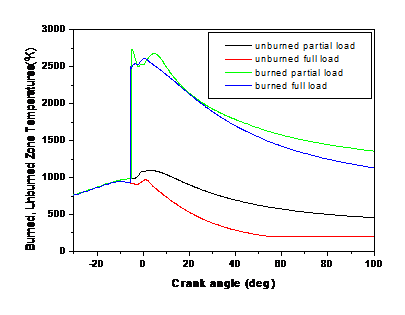Figure 6. Unburned and burned zone temperature versus crank angle

Figure 7 shows the variation of the total, convective, and radiation heat transfer rates versus crank angle for partial and full load at an engine speed of 2,000 rpm. The maximum values of the total, convective and radiation heat transfer are showed at 17° after TDC. When the mass full injected increased by 50%, the maximum total heat transfer value increases by 25%. It has also been noticed that the maximum convective heat transfer value increases by 16%, and the maximum radiation heat transfer value increase by 9%.

## 7.png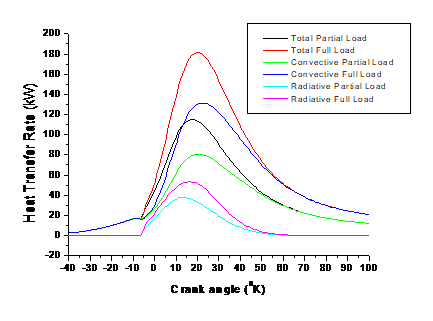Figure 7. Effect of engine load on total, convective and radiative heat transfer rate versus crank angle

## 8.pngFigure 8. Effect of engine load on heat flux versus crank angle

Figure 8 gives the evolution of heat flux from gas to cylinder wall versus crank angle, and for low, partial, and full loads at a constant engine speed of 2,000 rpm. The increase in the engine load causes a high peak in the rate of heat generation, the maximum values of heat flux are shown at 23°, 9° and 5° after TDC for low, partial and full load respectively. If the mass full injected increased by 50% (from partial to full load), the maximum heat flux value increases by 11%.

Figure 9 gives the variation with the crank angle of the in-cylinder simulated gas heat transfer coefficient, for various loads at an engine speed of 2,000 rpm and an injection timing angle of 15 BTDC. The heat transfer coefficient increases with increasing engine load. This is maybe due to the higher in-cylinder temperatures during the combustion period, where the heat transfer coefficient reaches its maximum value . The maximum values of the heat transfer coefficient are showed at 15°, 9°, and 7° after TDC for low, partial and full load. The maximum heat transfer coefficient value would increase by 20% by increasing the mass full injected by 50% (from partial to full load).

## 9.png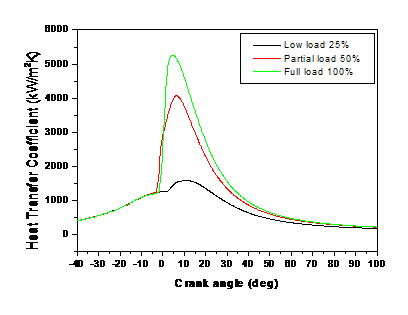Figure 9. Effect of engine load on the simulated gas heat transfer coefficient versus crank angle

The rate of heat release in energy per degree with the crank angle for different mass fuel injected (low, partial, and full load) for diesel engine running at 2,000 rpm is represented in Figure 10. The heat lost through convection increased due to the more injected fuel available for the conversion of chemical energy to heat energy.

## 10.pngFigure 10. Effect of engine load on the heat release rate versus crank angle

Figure 11 shows the influence of engine loads on the amount of fuel burned per unit crank angle. These results indicate that the burned fuel fraction increases with the crank angle in the dual-zone combustion model. The burnt gases’ volume increases during the combustion period; on the other hand, the fresh gases gradually decreases and is cancelled out at the end of combustion. The burned fuel mass fraction increases with the mass of the fuel injected into the combustion chamber to reach its maximum value at the end of this phase, which means that all the mass of the admitted fuel is burned (No; the curves indicates that only 40% is burnt for low load and 60% for partial load, for partial load the burnt mass is higher due to availably of oxygen and the good oxygen-fuel mixture, then stabilize at this level during the expansion period.

## 11.png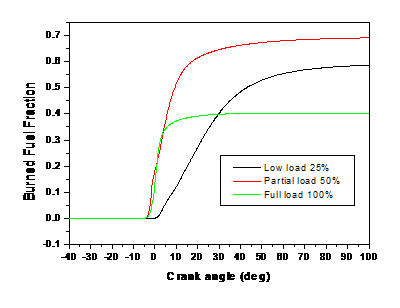Figure 11. Effect of engine load on burned fraction versus crank angle

Figure 12 shows the evolution of internal energy and work versus crank angle for partial and full load at engine speed of 2,000 rpm. More fuel in the combustion chamber causes more energy and work to be released, and thus the engine provides more power to the vehicle. The internal energy and work increases in the combustion phase due to the increase of injected fuel. An increase in the mass full injected by 50% would increase the maximum internal energy value by 50% while the maximum work value increases by 10%.

## 12.png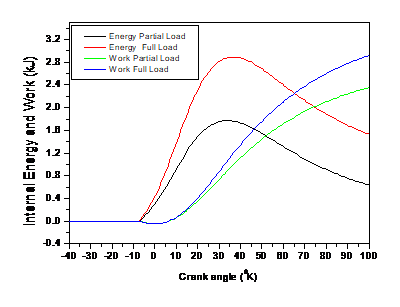Figure 12. Effect of engine load on internal energy and work versus crank angle

5. Conclusions

In this paper, a simulated dual-zone thermodynamic cycle model of a single-cylinder DI diesel engine is developed. The dual-zone combustion model developed in this study has shown its effectiveness in predicting the main combustion performance of the engine. Specifically, the effect of the major operating parameter of the load upon cylinder pressures and temperature, convective and radiation heat transfer, in-cylinder energy, and work. Based on the computational studies in predicting the heat transfer of the diesel engine, it can be concluded that:

• The comparison of the results obtained by the simulation tests, with those drawn from the bibliographical references, showed an acceptable agreement. With the parametric study, we could conclude that the calculation program is valid.
• The convective heat flux from the working gases to the cylinder wall varies during the working cycle from small negative values at -180° to -100° during compression to positive values after -100° and arrived maximal value at 23° after TDC.
• The radiation heat flux from the working gases to the walls varies during the combustion process and arrived maximal value at 23° after TDC.
• The increase of engine load entails a great increase of head peak heat flux from gas to the cylinder wall.

It can be concluded that the dual-zone combustion model is used to predict the main combustion performance of a DI diesel engine. In the future, the authors will try to treat the multi-zone combustion model taking into account the crevice losses and the propagation of heat between these zones.

Acknowledgment

This study was performed under the framework of ‘Projet Impact Socio-Economique’ Contract No. 362 funded by the General Directorate of Algerian Scientific Research (DGRSDT).

Nomenclature
 m mass gas in the combustion chamber, kg V instantaneous cylinder volume, m3 x in-cylinder burned mass fraction v specific volume of the system, m3 C blow-by coefficient h Enthalpy, J.kg-1 A surfaces of the gases, m2 T temperature, K BTDC befor top dead center ABDC after bottom dead center BBDC befor bottom dead center ATDC after top dead center Greek symbols θ crank angle, deg Subscripts b burned u unburned wall cylinder wall gr reference gas d displacement m motoring
References

 Lounici, M.S., Loubar, K., Balistrou, M., Tazerout, M. (2011). Investigation on heat transfer evaluation for a more efficient two-zone combustion model in the case of natural gas SI engines. Applied Thermal Engineering. 31(2-3): 319-328. http://dx.doi.org/10.1016/j.applthermaleng.2010.09.012

 Menacer, B., Bouchetara, M. (2014). Parametric study of the performance of a turbocharged compression ignition engine. Simulation, 90(12): 1375-1384. https://doi.org/10.1177/0037549714557046

 Ma, T., Feng, L., Wang, H., Liu, H.F., Yao, M.F. (2019). Analysis of near wall combustion and pollutant migration after spray impingement. International Journal of Heat and Mass Transfer, 141: 569-579. https://doi.org/10.1016/j.ijheatmasstransfer.2019.07.001

 Rakopoulos, D.C., Rakopoulos, C.D., Giakoumis, E.G., Papagiannakis, R.G. (2018). Evaluating oxygenated fuel’s influence on combustion and emissions in diesel engines using a two-zone combustion model. Journal of Energy Engineering, 144(4): 04018046. https://doi.org/10.1061/(ASCE)EY.1943-7897.0000556

 Liu, Y., Reitz, R.D. (1998). Modeling of heat conduction within chamber walls for multidimensional internal combustion engine simulations. International Journal of Heat and Mass Transfer, 41(6): 859-869. https://doi.org/10.1016/S0017-9310(97)00178-6

 Im, Y.H., Huh, K.Y. (2000). Phenomenological combustion modeling of a direct injection diesel engine with incylinder flow effects. KSME International Journal, 14(5): 569-581. https://doi.org/10.1007/BF03185660

 Mohammed, S.E., Baharom, M.B., A. Aziz, A.R., Zainal A., E.Z. (2019). Modelling of combustion characteristics of a single curved-cylinder spark-ignition crank-rocker engine. Energies, 12(17): 3313. https://doi.org/10.3390/en12173313

 Rakopoulos, C.D., Rakopoulos, D.C., Kyritsis, D.C. (2003). Development and validation of a comprehensive two-zone model for combustion and emissions formation in a DI diesel engine. International Journal of Energy Research. 27(14): 1221-1249. https://doi.org/10.1002/er.939

 Juntarakod, P., Soontornchainacksaeng, T. (2014). A quasi-dimensional three-zone combustion model of the diesel engine to calculate performances and emission using the diesel-ethanol dual fuel. Contemporary Engineering Sciences, 7(1): 19-37. http://dx.doi.org/10.12988/ces.2014.3951

 Ahmed, S.A., Zhou, S., Zhu, Y.Q., Tsegay, A.S., Feng, Y., Ahmad, N., Malik, A. (2020). Effects of pig manure and corn straw generated biogas and methane enriched biogas on performance and emission characteristics of dual fuel diesel engines. Energies, 13(4): 889. https://doi.org/10.3390/en13040889

 Arsie, I., Pianese, C., Sorrentino, M. (2007). Control parameters optimization in automotive diesel engines via two zone modelling. IFAC Proceedings Volumes, 40(10): 425-432. https://doi.org/10.3182/20070820-3-US-2918.00058

 Ollivier, E. (2006). Contribution to the characterization of heat transfer in spark ignition engines, application to knock detection. PhD thesis, ENSTIM de Nantes, France.

 Descieux, D., Feidt, M. (2007). One zone thermodynamic model simulation of an ignition compression engine. Applied Thermal Engineering, 27(8-9): 1457-1466. https://doi.org/10.1016/j.applthermaleng.2006.10.002

 Stull, D., Prophet, H. (1971). JANAF Thermochemical Tables, NSRDS-NBS 37. National Bureau of Standards, Washington, DC. http://purl.access.gpo.gov/GPO/LPS112842

 Ferguson, C.R., Kirkpatrick, A.T. (2015). Internal Combustion Engines: Applied Thermosciences. John Wiley & Sons.

 Xiang, L., Song, E., Ding, Y. (2018). A two-zone combustion model for knocking prediction of marine natural gas SI engines. Energies, 11(3): 561. https://doi.org/10.3390/en11030561

 Olikara, C., Borman, G.L. (1975). A computer program for calculating properties of equilibrium combustion products with some applications to IC engines. SAE Technical Paper. https://doi.org/10.4271/750468

 Shi, C., Ji, C.W., Wang, S.F., Yang, J.X., Ma, Z.D., Xu, P.Y. (2020). Assessment of spark-energy allocation and ignition environment on lean combustion in a twin-plug Wankel engine. Energy Conversion and Management, 209: 112597. https://doi.org/10.1016/j.enconman.2020.112597

 Mahabadipour, H., Srinivasan, K.K., Krishnan, S.R. (2019). An exergy analysis methodology for internal combustion engines using a multi-zone simulation of dual fuel low temperature combustion. Applied Energy, 256: 113952. https://doi.org/10.1016/j.apenergy.2019.113952

 Feng, Y., Du, Z.Q., Shreka, M., Zhu, Y.Q., Zhou, S., Zhang, W.P. (2020). Thermodynamic analysis and performance optimization of the supercritical carbon dioxide Brayton cycle combined with the Kalina cycle for waste heat recovery from a marine low-speed diesel engine. Energy Conversion and Management, 206: 112483. https://doi.org/10.1016/j.enconman.2020.112483

 Naima, K., Liazid, A., Tazerout, M., Bousbaa, H. (2018). Experimental and numerical investigation of combustion behaviour in diesel engine fuelled with waste polyethylene oil. Journal of Engineering Science and Technology, 13(10): 3204-3219.

 Pulkrabek, W.W. (2015). Engineering Fundamentals of the Internal Combustion Engine. 2nd edition, Pearson.

  Fonseca, L., Olmeda, P., Novella, R., Valle, R.M. (2019). Internal combustion engine heat transfer and wall temperature modeling: An overview. Archives of Computational Methods in Engineering, 27: 1661-1679. https://doi.org/10.1007/s11831-019-09361-9

 Yue, Z., Reitz, R.D. (2019). Numerical investigation of radiative heat transfer in internal combustion engines. Applied Energy, 235: 147-163. https://doi.org/10.1016/j.apenergy.2018.10.098

 Heywood, J.B. (2018). Internal Combustion Engine Fundamentals. 2nd edition, McGraw-Hill Education.

 Na, S. (2015). Correlation between cylinder pressure and noise emissions from diesel engines. Journal of KONES Powertrain and Transport, 22(1): 243-254. https://doi.org/10.5604/12314005.1161775